# Extended analysis of sensor- and source-level connectivity

## Introduction

In this tutorial we will explore different measures of connectivity, using simulated data and using source-level MEG data. You will learn how to compute various connectivity measures and how these measures can be interpreted. Furthermore, a number of interpretational problems will be addressed.

Since this tutorial focusses on connectivity measures in the frequency domain, we expect that you understand the basics of frequency domain analysis, because this is not covered by this tutorial.

Note that this tutorial does not cover all possible pitfalls associated with the analysis of connectivity and the interpretational difficulties. Although the computation of connectivity measures might be easy using FieldTrip, the interpretation of the outcomes of those measures in terms of brain networks and activity remains challenging and should be exercised with caution.

## Background

The brain is organized in functional units, which at the smallest level consists of neurons, and at higher levels consists of larger neuronal populations. Functional localization studies consider the brain to be organized in specialized neuronal modules corresponding to specific areas in the brain. These functionally specialized brain areas (e.g., visual cortex area V1, V2, V4, MT, …) have to pass information back and forth along anatomical connections. Identifying these functional connections and determining their functional relevance is the goal of connectivity analysis and can be done using ft_connectivityanalysis and associated functions.

The nomenclature for connectivity analysis can be adopted from graph theory, in which brain areas correspond to nodes or vertices and the connections between the nodes is given by edges. One of the fundamental challenges in the analysis of brain networks from MEG and EEG data lies not only in identifying the “edges”, i.e. the functional connections, but also the “nodes”. The remainder of this tutorial will only explain the methods to characterize the edges, but will not go into details for identifying the nodes. The beamformer tutorial and corticomuscular coherence tutorial provide more pointers to localizing the nodes.

Many measures of connectivity exist, and they can be broadly divided into measures of functional connectivity (denoting statistical dependencies between measured signals, without information about causality/directionality), and measures of effective connectivity, which describe directional interactions. If you are not yet familiar with the range of connectivity measures (i.e., mutual information, coherence, granger causality), you may want to read through some of the papers listed under the method references for connectivity analysis

After the identification of the network nodes and the characterization of the edges between the nodes, it is possible to analyze and describe certain network features in more detail. This network analysis is also not covered in this tutorial, although FieldTrip provides some functionality in this direction (see ft_networkanalysis to get started).

## Procedure

This tutorial consists of three part

• Simulated data with directed connections. In this part we are going to simulate some data and use these data to compute various connectivity metrics. As a generative model of the data we will use a multivariate autoregressive model and we will use ft_connectivitysimulation for this. Subsequently, we will estimate the multivariate autoregressive model and the spectral transfer function, and the cross-spectral density matrix using the functions ft_mvaranalysis and ft_freqanalysis. In the next step we will compute and inspect various measures of connectivity with ft_connectivityanalysis and ft_connectivityplot.
• Simulated data with common pick-up and different noise levels. In this part we are going to simulate some data consisting of an instantaneous mixture of 3 ‘sources’, creating a situation of common pick up. We will explore the effect of this common pick up on the consequent estimates of connectivity, and we will investigate the effect of different mixings on these estimates.
• Connectivity between MEG virtual channels and EMG. In this part we are going to reconstruct MEG virtual channel data and estimate connectivity between these virtual channels and EMG. The data used for this part are the same as in the extended beamforming tutorial.

## Simulated data with directed connections

We will first simulate some data with a known connectivity structure built in. This way we know what to expect in terms of connectivity. To simulate data we use ft_connectivitysimulation. We will use an order 2 multivariate autoregressive model. The necessary ingredients are a set of NxN coefficient matrices, one matrix for each time lag. These coefficients need to be stored in the cfg.param field. Next to the coefficients we have to specify the NxN covariance matrix of the innovation noise. This matrix needs to be stored in the cfg.noisecov field.

The model we are going to use to simulate the data is as follow

x(t) = 0.8x(t-1) - 0.5x(t-2)

y(t) = 0.9y(t-1) + 0.5z(t-1) - 0.8*y(t-2)

z(t) = 0.5z(t-1) + 0.4x(t-1) - 0.2*z(t-2)

which is done using

``````% always start with the same random numbers to make the figures reproducible
rng default
rng(50)

cfg             = [];
cfg.ntrials     = 500;
cfg.triallength = 1;
cfg.fsample     = 200;
cfg.nsignal     = 3;
cfg.method      = 'ar';

cfg.params(:,:,1) = [ 0.8    0    0 ;
0  0.9  0.5 ;
0.4    0  0.5];

cfg.params(:,:,2) = [-0.5    0    0 ;
0 -0.8    0 ;
0    0 -0.2];

cfg.noisecov      = [ 0.3    0    0 ;
0    1    0 ;
0    0  0.2];

data              = ft_connectivitysimulation(cfg);
``````

The simulated data consists of 3 channels (cfg.nsignal) in 500 trials (cfg.ntrials). You can easily visualize the data for example in the first trial using

``````figure
plot(data.time{1}, data.trial{1})
legend(data.label)
xlabel('time (s)')
``````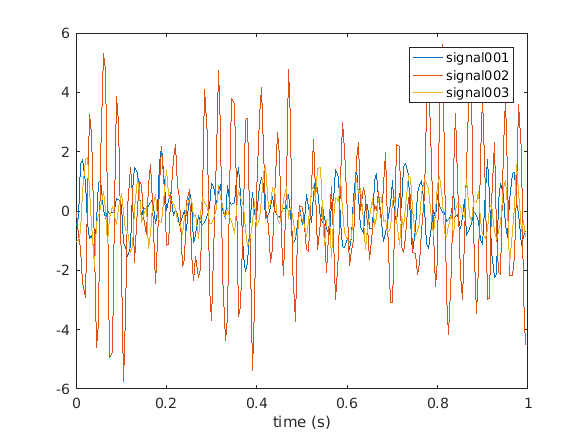or browse through the complete data using

``````cfg = [];
cfg.viewmode = 'vertical';  % you can also specify 'butterfly'
ft_databrowser(cfg, data);
``````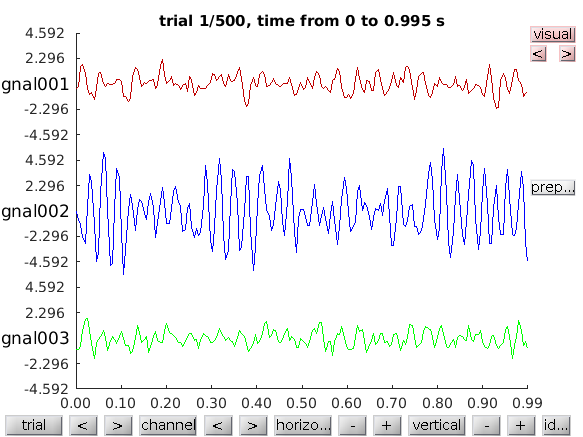### Computation of the multivariate autoregressive model

To be able to compute spectrally resolved Granger causality, or other frequency-domain directional measures of connectivity, we need to estimate two quantities: the spectral transfer matrix and the covariance of an autoregressive model’s residuals. We fit an autoregressive model to the data using the ft_mvaranalysis function.

For the actual computation of the autoregressive coefficients FieldTrip makes use of an implementation from third party toolboxes. At present ft_mvaranalysis supports the biosig and bsmart toolboxes for these computations.

In this tutorial we will use the bsmart toolbox. The relevant functions have been included in the FieldTrip release in the fieldtrip/external/bsmart directory. Although the exact implementations within the toolboxes differ, their outputs are comparable.

``````cfg         = [];
cfg.order   = 5;
cfg.toolbox = 'bsmart';
mdata       = ft_mvaranalysis(cfg, data);

mdata =
dimord: 'chan_chan_lag'
label: {3x1 cell}
coeffs: [3x3x5 double]
noisecov: [3x3 double]
dof: 500
fsampleorig: 200
cfg: [1x1 struct]
``````

The resulting variable mdata contains a description of the data in terms of a multivariate autoregressive model. For each time-lag up to the model order (cfg.order), a 3x3 matrix of coefficients is outputted. The noisecov-field contains covariance matrix of the model’s residuals.

Here, we know the model order a priori because we simulated the data and we choose a slightly higher model order (five instead of two) to get more interesting results in the output. For real data the appropriate model order for fitting the autoregressive model can vary depending on subject, experimental task, quality and complexity of the data, and model estimation technique that is used. You can estimate the optimal model order for your data by relying on information criteria methods such as the Akaike information criterion or the Bayesian information criterion. Alternatively, you can choose to use a non-parametric approach without having to decide on model order at all (see next section on Non-parametric computation of the cross-spectral density matrix)

#### Exercise 1

Compare the parameters specified for the simulation with the estimated coefficients and discuss.

### Computation of the spectral transfer function

From the autoregressive coefficients it is now possible to compute the spectral transfer matrix, for which we use ft_freqanalysis. There are several ways of computing the spectral transfer function, the parametric and the non-parametric way. We will first illustrate the parametric route:

``````cfg        = [];
cfg.method = 'mvar';
mfreq      = ft_freqanalysis(cfg, mdata);

mfreq =

freq: [1x101 double]
transfer: [3x3x101 double]
noisecov: [3x3 double]
dof: 500
label: {3x1 cell}
dimord: 'chan_chan_freq'
cfg: [1x1 struct]
``````

The resulting mfreq data structure contains the pairwise transfer function between the 3 channels for 101 frequencies.

It is also possible to compute the spectral transfer function using non-parametric spectral factorization of the cross-spectral density matrix. For this, we need a Fourier decomposition of the data. This is done in the following section.

### Non-parametric computation of the cross-spectral density matrix

Some connectivity metrics can be computed from a non-parametric spectral estimate (i.e. after the application of the FFT-algorithm and conjugate multiplication to get cross-spectral densities), such as coherence, phase-locking value and phase slope index. The following part computes the Fourier-representation of the data using ft_freqanalysis.

``````cfg           = [];
cfg.method    = 'mtmfft';
cfg.taper     = 'dpss';
cfg.output    = 'fourier';
cfg.tapsmofrq = 2;
freq          = ft_freqanalysis(cfg, data);

freq =
label: {3x1 cell}
dimord: 'rpttap_chan_freq'
freq: [1x101 double]
fourierspctrm: [1500x3x101 double]
cumsumcnt: [500x1 double]
cumtapcnt: [500x1 double]
cfg: [1x1 struct]
``````

The resulting freq structure contains the spectral estimate for 3 tapers in each of the 500 trials (hence 1500 estimates), for each of the 3 channels and for 101 frequencies. It is not necessary to compute the cross-spectral density at this stage, because the function used in the next step, ft_connectivityanalysis, contains functionality to compute the cross-spectral density from the Fourier coefficients.

We apply frequency smoothing of 2Hz. The tapsmofrq parameter should already be familiar to you from the multitapers section of the frequency analysis tutorial. How much smoothing is desired will depend on your research question (i.e. frequency band of interest) but also on whether you decide to use the parametric or non-parametric estimation methods for connectivity analysis:

Parametric and non-parametric estimation of Granger causality yield very comparable results, particularly in well-behaved simulated data. The main advantage in calculating Granger causality using the non-parametric technique is that it does not require the determination of the model order for the autoregressive model. When relying on the non-parametric factorization approach more data is required as well as some smoothing for the algorithm to converge to a stable result. Thus the choice of parametric vs. non-paramteric estimation of Granger causality will depend on your data and your certainty of model order. (find this information and more in Bastos and Schoffelen 2016)

### Computation and inspection of the connectivity measures

The actual computation of the connectivity metric is done by ft_connectivityanalysis. This function is transparent to the type of input data, i.e. provided the input data allows the requested metric to be computed, the metric will be calculated. Here, we provide an example for the computation and visualization of the coherence coefficient.

``````cfg           = [];
cfg.method    = 'coh';
coh           = ft_connectivityanalysis(cfg, freq);
cohm          = ft_connectivityanalysis(cfg, mfreq);
``````

Subsequently, the data can be visualized using ft_connectivityplot.

``````cfg           = [];
cfg.parameter = 'cohspctrm';
cfg.zlim      = [0 1];
ft_connectivityplot(cfg, coh, cohm);
``````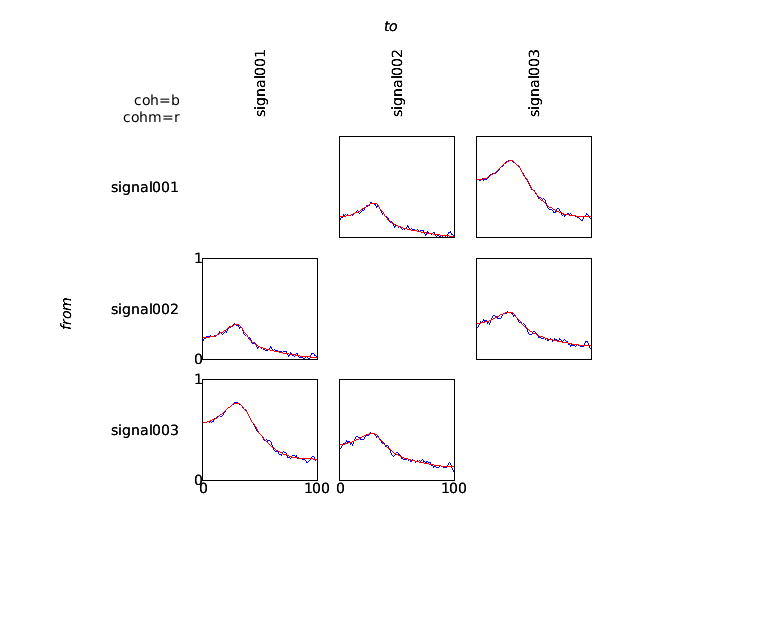The coherence measure is a symmetric measure, which means that it does not provide information regarding the direction of information flow between any pair of signals. In order to analyze directionality in interactions, measures based on the concept of granger causality can be computed. These measures are based on an estimate of the spectral transfer matrix, which can be computed in a straightforward way from the multivariate autoregressive model fitted to the data.

``````cfg           = [];
cfg.method    = 'granger';
granger       = ft_connectivityanalysis(cfg, mfreq);

cfg           = [];
cfg.parameter = 'grangerspctrm';
cfg.zlim      = [0 1];
ft_connectivityplot(cfg, granger);
``````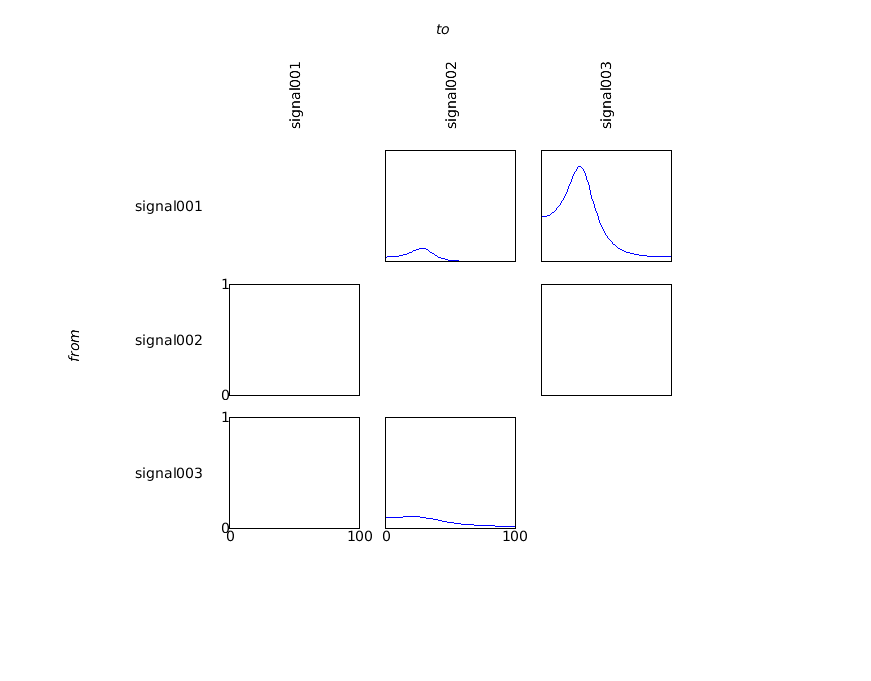#### Exercise 2

Compute the granger output using instead the ‘freq’ data structure. Plot them side-by-side using ft_connectivityplot.

Instead of plotting it with ft_connectivityplot, you can use the following low-level MATLAB plotting code which gives a better understanding of the numerical representation of the results.

``````figure
for row=1:3
for col=1:3
subplot(3,3,(row-1)*3+col);
plot(granger.freq, squeeze(granger.grangerspctrm(row,col,:)))
ylim([0 1])
end
end
``````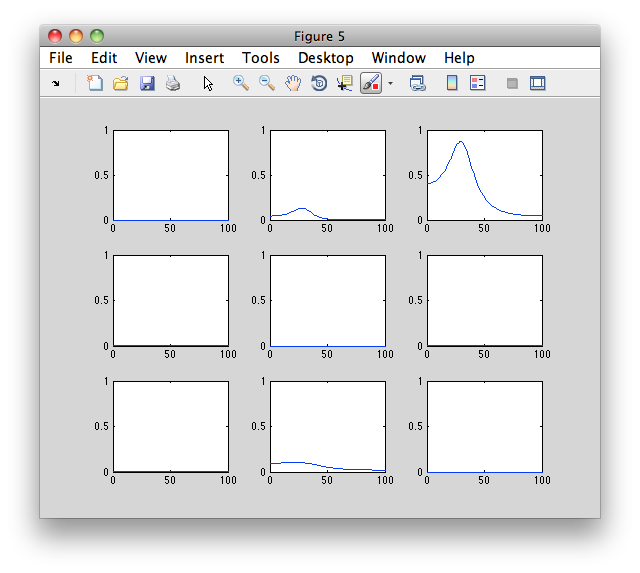#### Exercise 3

Discuss the differences between the granger causality spectra, and the coherence spectra.

#### Exercise 4

Compute the following connectivity measures from the mfreq data, and visualize and discuss the results: partial directed coherence (pdc), directed transfer function (dtf), phase slope index (psi). (Note that psi will require specifying cfg.bandwidth. What is the meaning of this parameter?)

## Computation of virtual MEG channels in source-space

In the extended beamformer tutorial we identified two potentially interesting regions, one which produces visual gamma-band activity and the other which is coherent with the EMG sensors. If you want to continue analyzing those two regions it is pretty unhandy to juggle around with the two source structures all the time. Also, using the DICS method you do not get a time-resolved signal of these sources. In the following example we will show how you can create virtual channels out of these two sources, which can then be used for further analysis, for example connectivity analysis.

### Compute the spatial filter for the region of interest

After having done all steps in the extended beamformer tutorial, you have the preprocessed data, two source structures, and a headmodel. You can also get these from the download server:

We will now determine the positions on which the cortico-muscular coherence is the largest and the position where the induced visual gamma activity is largest:

``````[maxval, maxcohindx] = max(source_coh_lft.avg.coh);
source_coh_lft.pos(maxcohindx, :)

ans =
3.2000   -0.6000   7.4000

[maxval, maxpowindx] = max(source_diff.avg.pow);
source_diff.pos(maxpowindx, :)

ans =
0.4000   -8.8000    2.6000
``````

The cortical position is expressed in MNI space according to the template brain we used for warping and in centimeter. Relative to the anterior commissure (AC) the coherence peak position is 3.2 cm towards the right side of the brain, -0.6 towards the front of the AC (i.e., 0.6 cm towards the back!) and 7.4 cm towards the vertex. The visual gamma peak is 0.4 cm towards the right of the brain , -8.8 cm to the front of the AC (i.e. 8.6 cm to the back) and 2.6 cm to the top.

The ft_sourceanalysis methods are usually applied to the whole brain using a regular 3-D grid or using a triangulated cortical sheet. You can also just specify the location of a single or multiple points of interest with cfg.sourcemodel.pos and the LCMV beamformer will simply be performed at the location of interest. Note that we have to use subject-specific coordinates here and not the MNI template.

The LCMV beamformer spatial filter for the location of interest will pass the activity at that location with unit-gain, while optimally suppressing all other noise and other source contributions to the MEG data. The LCMV implementation in FieldTrip requires the data covariance matrix to be computed with ft_timelockanalysis.

``````cfg                   = [];
cfg.covariance        = 'yes';
cfg.channel           = 'MEG';
cfg.vartrllength      = 2;
cfg.covariancewindow  = 'all';
tlock = ft_timelockanalysis(cfg, data_cmb);

cfg                     = [];
cfg.method              = 'lcmv';
cfg.sourcemodel.pos     = sourcemodel.pos([maxcohindx maxpowindx], :);
cfg.sourcemodel.inside  = true(2,1);
cfg.unit                = sourcemodel.unit;
cfg.lcmv.keepfilter     = 'yes';
source_idx = ft_sourceanalysis(cfg, tlock);
``````

The source reconstruction contains the estimated power and the source-level time series of the averaged ERF, but here we are not interested in those. The cfg.keepfilter option results in the spatial filter being kept in the output source structure. That spatial filter can be used to reconstruct the single-trial time series as a virtual channel by multiplying it with the original MEG data.

### Extract the virtual channel time series

``````beamformer_lft_coh = source_idx.avg.filter{1};
beamformer_gam_pow = source_idx.avg.filter{2};

chansel  = ft_channelselection('MEG', data_cmb.label); % find MEG sensor names
chanindx = match_str(data_cmb.label, chansel);         % find MEG sensor indices

coh_lft_data = [];
coh_lft_data.label = {'coh_lft_x', 'coh_lft_y', 'coh_lft_z'};
coh_lft_data.time = data_cmb.time;

gam_pow_data = [];
gam_pow_data.label = {'gam_pow_x', 'gam_pow_y', 'gam_pow_z'};
gam_pow_data.time  = data_cmb.time;

for i=1:length(data_cmb.trial)
coh_lft_data.trial{i} = beamformer_lft_coh * data_cmb.trial{i}(chanindx,:);
gam_pow_data.trial{i} = beamformer_gam_pow * data_cmb.trial{i}(chanindx,:);
end
``````

The LCMV spatial filter is computed here without applying any time-domain filters. Consequently, it will have to suppress all noise in the data in all frequency bands. The spatial filter derived from the broadband data allows us to compute a broadband source level time series.

If you would know that the subsequent analysis would be limited to a specific frequency range in the data (e.g., everything above 30 Hz), you could first apply a filter using ft_preprocessing (e.g., cfg.hpfilter=yes and cfg.hpfreq=30) prior to computing the covariance and the spatial filter.

The structures coh_lft_data and gam_pow_data resemble the raw-data output of ft_preprocessing and consequently can be used in any follow-up function. You can for example visualize the single-trial virtual channel time series using ft_databrowser.

``````cfg = [];
cfg.viewmode = 'vertical';  % you can also specify 'butterfly'
ft_databrowser(cfg, gam_pow_data);
``````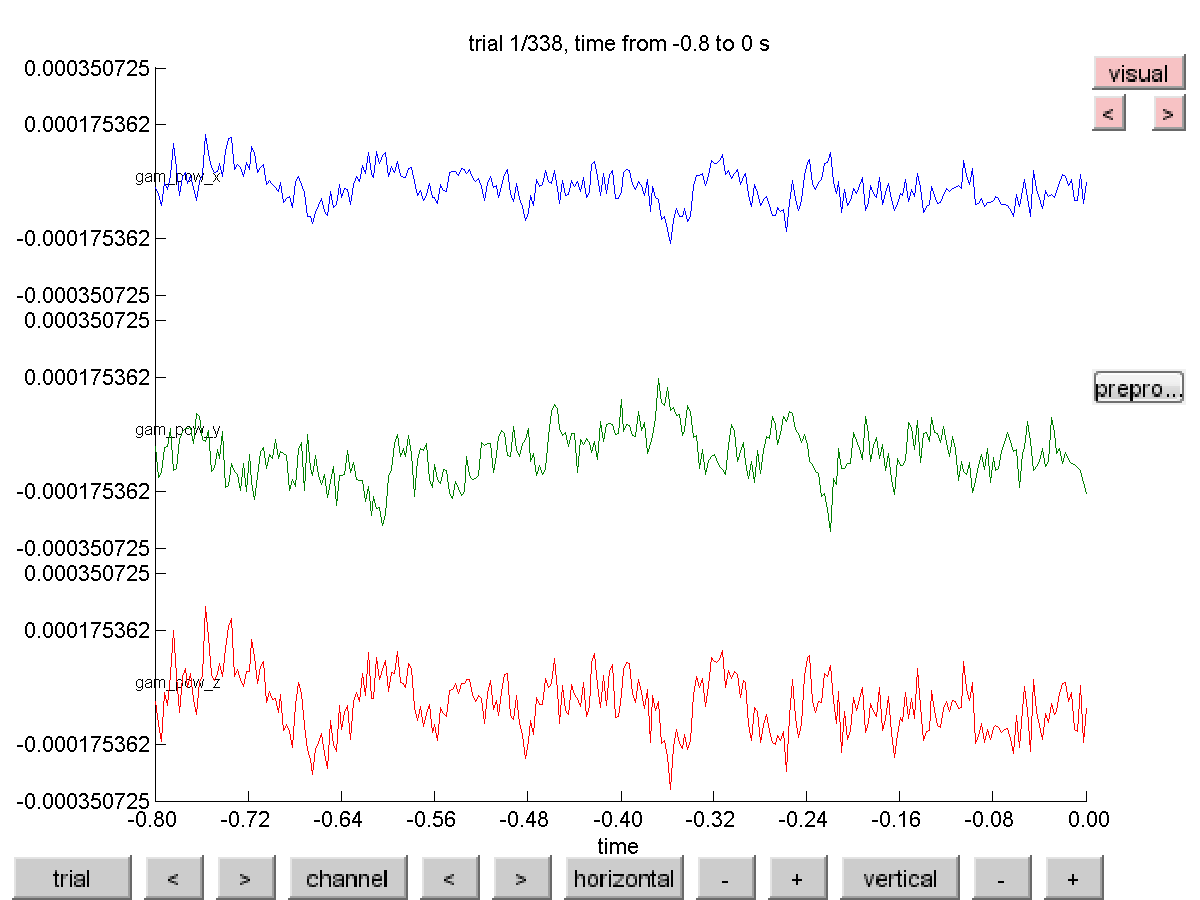Notice that the reconstruction contains three channels, for the x-, the y- and the z-component of the equivalent current dipole source at the location of interest.

### Project along the strongest dipole direction

The virtual channel data just computed has three channels per location. These correspond to the three orientations of the dipole in a single voxel. The interpretation of connectivity is facilitated if we can compute it between plain channels rather than between triplets of channels. Therefore we will project the time series along the dipole direction that explains most variance. This projection is equivalent to determining the largest (temporal) eigenvector and can be computationally performed using the singular value decomposition (svd).

``````visualTimeseries = cat(2, gam_pow_data.trial{:});
motorTimeseries = cat(2, coh_lft_data.trial{:});
[u1, s1, v1] = svd(visualTimeseries, 'econ');
[u2, s2, v2] = svd(motorTimeseries, 'econ');
``````

Matrices u1 and u2 contain the spatial decomposition, matrices v1 and v2 the temporal and on the diagonal of matrices s1 and s2 you can find the eigenvalues. See “help svd” for more details.

We now recompute the virtual channel time series, but now only for the dipole direction that has the most power.

``````virtualchanneldata = [];
virtualchanneldata.label = {'visual', 'motor'};
virtualchanneldata.time = data_cmb.time;

for k = 1:length(data_cmb.trial)
virtualchanneldata.trial{k}(1,:) = u1(:,1)' * beamformer_gam_pow * data_cmb.trial{k}(chansel,:);
virtualchanneldata.trial{k}(2,:) = u2(:,1)' * beamformer_lft_coh * data_cmb.trial{k}(chansel,:);
end
``````

### Combine the virtual channel with the EMG

The raw data structure containing one (virtual) channel can be combined with the two EMG channels from the original preprocessed data.

``````% select the two EMG channels
cfg = [];
cfg.channel = 'EMG';
emgdata = ft_selectdata(cfg, data_cmb);

% combine the virtual channel with the two EMG channels
cfg = [];
combineddata = ft_appenddata(cfg, virtualchanneldata, emgdata);
``````

### Compute the connectivity

The resulting combined data structure now has four channels: the activity from the visual cortex, the activity from the right motor cortex, the left EMG and the right EMG. We can now treat this data structure as any other, and perform connectivity analysis ‘as if’ we were working on a channel-level data set!

``````%% compute the spectral decomposition
cfg            = [];
cfg.output     = 'fourier';
cfg.method     = 'mtmfft';
cfg.foilim     = [5 100];
cfg.tapsmofrq  = 5;
cfg.keeptrials = 'yes';
cfg.channel    = {'visual' 'motor' 'EMGlft' 'EMGrgt'};
freq = ft_freqanalysis(cfg, combineddata);

cfg = [];
cfg.method = 'coh';
coherence = ft_connectivityanalysis(cfg, freq);
``````

This computes the spectral decomposition and the coherence spectrum between all channel pairs, which can be plotted with

``````cfg = [];
cfg.zlim = [0 0.25];
figure
ft_connectivityplot(cfg, coherence);
``````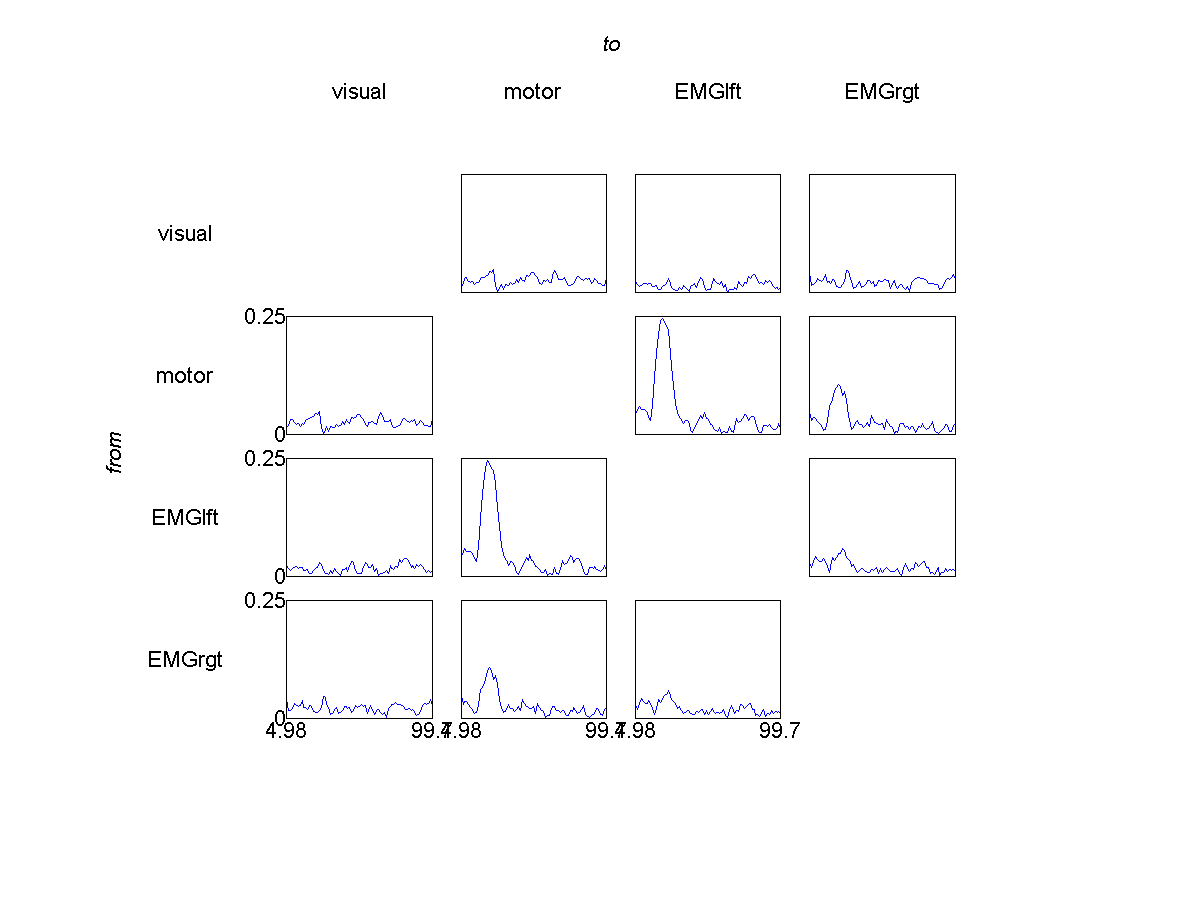The spectrum reveals a strong coherence peak around 20 Hz between the right motor cortex and the left EMG, as expected, and as we found in the beamforming tutorial as well, where we beamed the sensor-level coherence directly. Additionally, we also see a corticomuscular coherence peak in the gamma frequency range.

Rather than looking at undirected coherence, the virtual channel level data can now also easily be submitted to directed connectivity measures. Compute the spectrally resolved granger connectivity and try to assess whether the directionality is from cortex to EMG or vice versa.

Now that you have the virtual channel data, you can also use it to look at, for instance, power correlations across trials between visual gamma and motor beta. Do this! (Hint: this involves computing trial-specific estimates of power using ft_freqanalysis, extracting those estimates from the resulting freq structure, and using matlab’s own corr function.)